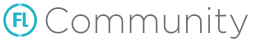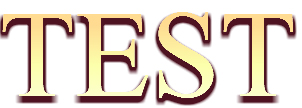# Gradient fill for TextField with TrueType font

Is it possible to create something like this without using bitmap fonts?
https://bit.ly/3bvEo8u

A viable solution since the glory days of flash goes a little bit like this.

• create a textfield with your desired text and give it a 90° rotated DropShadow filter
• create a duplicate of that textfield without the filter
• layer a rectangle shape the size of the textfield on top, filled with a linear gradient
• finally use the second textfield as a mask for the gradient shapeHere’s the code:

``````	var tFormat:TextFormat = new TextFormat(null, 124);

var tField:TextField = new TextField();
tField.defaultTextFormat = tFormat;
tField.textColor = 0xffffff;
tField.text = "TEST";
tField.width = 300;
tField.height = 200;

var colors:Array<UInt> = [0xffffff, 0xfcffab, 0xffdb91];
var alphas:Array<Float> = [1.0, 1.0,1.0];
var ratios:Array<Int> = [0x33, 0x99, 0xcc];
var mat:Matrix = new Matrix();
mat.createGradientBox(300, 200, -Math.PI / 2, 0, 0);
Unfortunately, I cannot draw result to BitmapData. Doesn’t work. Seems that sprite, that has textfield as mask cannot be drawn to bitmapdata